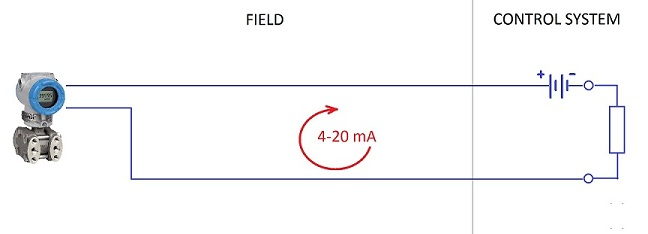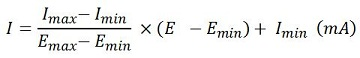## Current Loop Calculator >> PRESS HERE TO GO TO CALCULATORFree online application for the calculation of current analogue loops.

The purpose of 4-20mA loops is to transmit the information from sensors that are measuring physical parameters such as temperature, flow rate, pressure, speed, etc. It is an analog signal standard where the electric current is used to proportionately represent measurements. The physical signal is converted to a current signal where typically a 4mA current represents the sensor zero level output and 20mA represents the sensor’s full scale output. Any current value in between 4 and 20mA represents a percentage in between 0% and 100%.

Current loops are useful when the information has to be sent over long distances. Although long distances produce voltage losses due to the wiring resistance they do not reduce the 4-20mA current if the loop is correctly calculated.

Once the field instrument is calibrated the correspondence between maximum and minimum values of physical parameter and current are set.
The calculation is not difficult and for the calculation of the current in a 4-20mA loop the following formula can be used:or you can use the following calculator: 4-20mA loop calculator